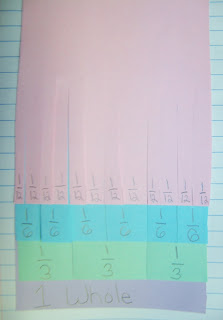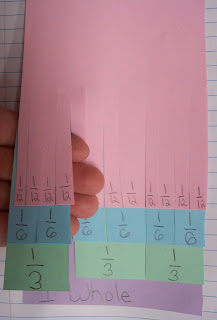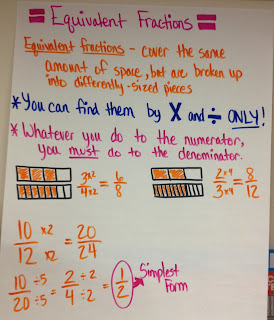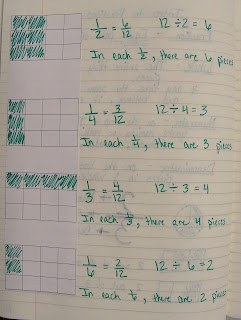## Thursday, November 29, 2012

### Mixed Numbers and Improper FractionsWe introduced fractions greater than one by modeling them with pattern blocks and writing them as a mixed number, and also as an improper fraction. Then we discussed the similarity between the two numbers.

After lots of examples with pattern blocks, we moved to pictures in our journals. We started with the mixed number, drew the picture, then named it as an improper fraction. After several of those, we started with the improper fraction, drew the picture, then named it as a mixed number.

The next day, we talked about how we went between the two types of fractions using pictures to help us. After examining our pictures, we noticed some shortcuts to help us be more efficient when changing from one type of fraction to the other. Eventually, the kids discovered the multiplication and division connection to help speed them along.Fractions Greater Than One Anchor Chart

## Friday, November 16, 2012

### Equivalent FractionsOur 1/2 fraction flipbook

We begin talking about equivalent fractions after we've had a lengthy discussion regarding the relationship between 1/2, 1/4, 1/8, 1/16. etc. as well as the relationship between 1/3, 1/6, 1/12, etc. The kids have a really good grasp on this idea.

We begin by making our fraction flipbooks (which takes a bit!) and then lifting up a fraction to see its equivalent fractions. For example, when we lift up 1/2, we can see it is equivalent to 2/4, 4/8, and 8/16. After we write down lots of equivalent fractions we find, we discuss their relationship. Finally, we discuss the multiplication and division involved. This is a great visual way for kids to come up with the relationships on their own!Flipbook showing fractions equivalent to 1/2Our 1/3 Fraction FlipbookFractions equivalent to 2/8Fractions equivalent to 1/3Our Equivalent Fraction Anchor ChartOur journal entry

## Friday, November 9, 2012

### Forms Of Energy Flip Book

As we discussed different forms of energy, (we focused on Mechanical, Sound, Electrical, Light/Solar, and Heat/Thermal) we decided to make a flip book to hold all of our information. Inside we put the definition of the form of energy, and added picture examples as well. Here are two examples of our flip books - one picture is the outside view and the other picture is inside a flap.

### Intro to FractionsFraction Basics

In math we have started to discuss fractions. By 4th grade, the kiddos have had a ton of practice naming fractions of a shape, as well as naming fractions of a set of objects. We continued that practice and discussed the definitions of the numerator and denominator. We've also extended our knowledge by figuring out how to find fractions of a number, such as 12 and 24.
We began by pulling out 12 squares and physically dividing them up into equal groups. It didn't take the kids long to figure out that to find fractions of a number, all they had to do was divide the number by the denominator of the fraction they were looking to find. For example, if they want to find 1/4 of 24, they could just divide 24 into 4 equal groups. Since 6 would be in each group, 1/4 of 24 is 6. Finally, we discussed how, if we found 1/4, we could also find 2/4, 3/4, etc.Finding fractions of 12 with blocksFinding fractions of 24Finding fractions of 24